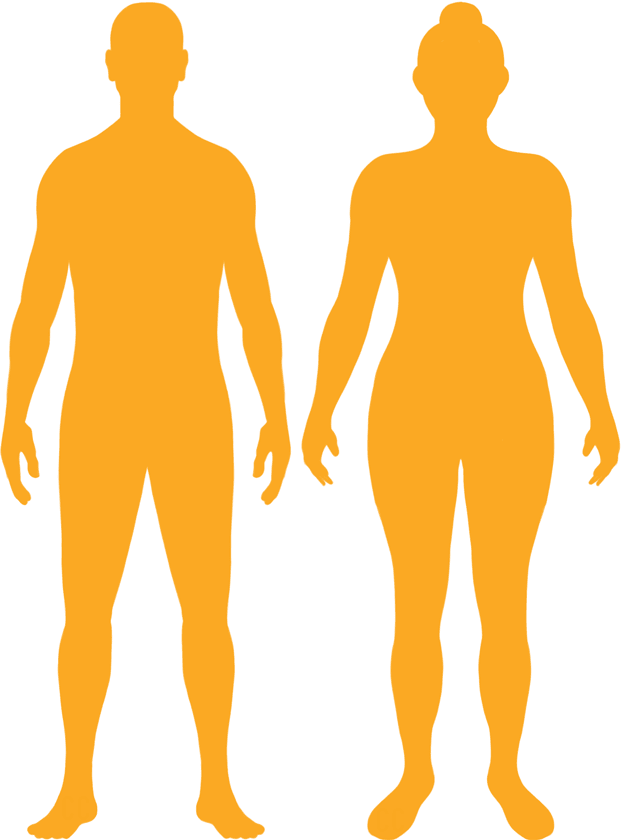BMI Calculator

Result for 231.14cm and 158.76kgs.
Change Here.29.72

Overweight BMI

At 231.14cm and 158.76kgs your BMI is 29.72

BMI Scale

 Under Normal Over Obese
-25 kgs
to reach a
normal BMI classification.
Normal BMI Ends:
133.6 kgs
+2 kgs
until you reach an obese BMI classification.
160.28 kgs

BMI Grading Table2 for Height 231.14cm

 Weight Weight Class Below 98.8 kgs Underweight 98.8 kgs - 133.6 kgs Normal Weight 133.6 kgs - 160.28 kgs Overweight 160.28 kgs and Above Obese

Weight Loss

If you were seeking a normal BMI weight of 133.6kgs, and you lost 0.90 kilos a week4, then you could reach a normal BMI in
27.5 Weeks

Overweight BMI SilhouetteIdeal Weight for a 231.14cm Male or Female2

The ideal weight range using the BMI for a male or female with a height of 231.14cm is

Between

98.8 kgs

and
133.6 kgs
How to Calculate BMI3 for 231.14cm and 158.76 kgs
158.76 / (2.3114 x 2.3114)
= 29.716035763432
231.14 = height | 158.76 = weight kgs

Conversion

• 231.14cm = 7 foot 7
• 231.14cm = 91 inches
• 158.76 kg = 350.01 pounds
• 158.76 kg = 25 stone 0 pounds
• 158.76 kg = 158760 grams

231.14cm and 158.76kg Summary

What is the BMI for a 231.14cm and 158.76kg female? 29.72 BMI, Overweight.
What is the BMI for a 231.14cm and 158.76kg male? 29.72 BMI, Overweight.
What is the ideal weight for a 231.14cm female? Between: 98.8kgs and 133.6kgs
What is the ideal weight for a 231.14cm male? Between: 98.8kgs and 133.6kgs
If I am 231.14cm and weigh 158.76kgs, is that a good weight for my height? Under the BMI classification, 158.76kgs is classed as being Overweight.